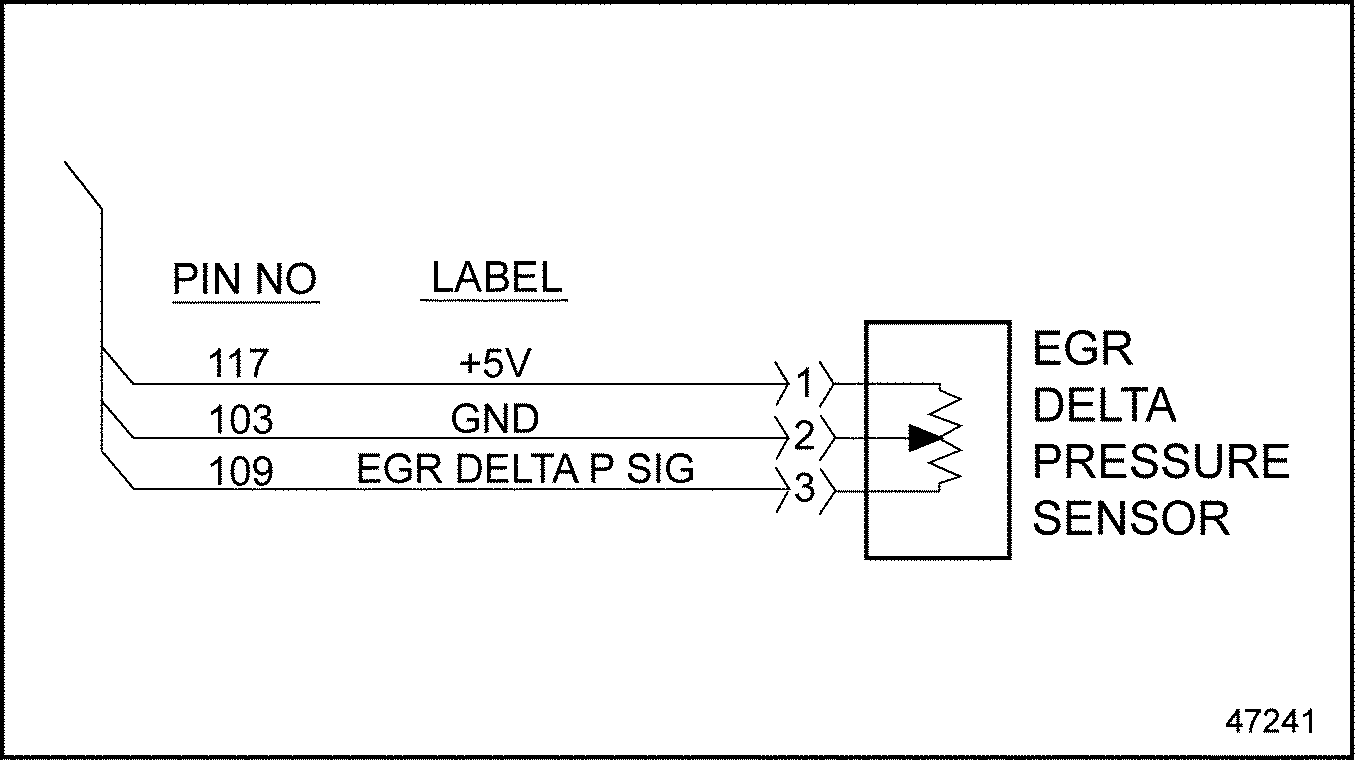# Section 39.3 SPN 411/FMI 4

### Section 39.3 SPN 411/FMI 4

This diagnosis is typically EGR Delta P Sensor short to ground.‪

#### Section 39.3.1 Short to Ground Check

Check as follows:‪

1. Disconnect the EGR Delta P Sensor.
2. Disconnect the 120–pin MCM connector.
3. Measure the resistance between pins 1 and 2 of the EGR Delta P Sensor.
1. If the resistance is greater than 5Ω, go to step 4 .
2. If the resistance is less than 5Ω, repair the short between pins 103 and 117 of the 120–pin MCM connector. Refer to "39.3.1.1 Verify Repairs" .
4. Measure the resistance between pins 2 and 3 of the EGR Delta P Sensor.
1. If the resistance is greater than 5Ω, go to step 5 .
2. If the resistance is less than 5Ω, repair the short between pins 103 and 109 of the 120–pin MCM connector. See Figure "EGR Delta P Sensor" . Refer to "39.3.1.1 Verify Repairs" .
3.Figure 1. EGR Delta P Sensor

5. Measure the resistance between pin 1 of the EGR Delta P Sensor and ground.
1. If the resistance is greater than 3Ω, go to step 6 .
2. If the resistance is less than 3Ω, repair the short circuit between pin 1 of the EGR Delta P Sensor harness connector and ground. Refer to "39.3.1.1 Verify Repairs" .
6. Measure the resistance between pin 3 of the EGR Delta P Sensor and ground.
1. If the resistance is greater than 3Ω, repeat steps 3 through 6 . If the results are the same, call the Detroit Diesel Customer Support Center (313–592–5800).
2. If the resistance is less than 3Ω, repair the short circuit between pin 3 of the EGR Delta P Sensor harness connector and ground. Refer to "39.3.1.1 Verify Repairs" .
##### Section 39.3.1.1 Verify Repairs

Verify repairs as follows:‪

1. Turn ignition OFF.
2. Reconnect any electrical connections that were disconnected to perform the diagnosis.